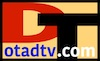Blog

#The Decibels (dB) Scale

The decibels (dB) scale is more convenient than a linear scale when dealing with signal loss or gain (dB), or power (dBm).

## dB - Decibel

Signal loss or gain is commonly measured in power decibels (dB), and is the power ratio of watts out / watts in, on a base 10 logarithmic scale. Minus three decibels (-3 dB) has a power ratio of about half, plus three decibels (3 dB) is almost double power. Signal loss is a negative (-) dB, signal gain is a positive (+) dB, 0 dB is no loss or gain (a ratio of 1/1).

= = dB 10 log10(ratio) ratio 10dB/10
 ratio = Pout / Pin Pout = Output signal power in watts. Pin = Input signal power in watts.
Convert dB to/from Ratio

Convert dB's and ratio

## dBm - Signal Power

Signal power is commonly measured in watts or dBm. A dBm is decibels above (+) or below (-) one milliwatt (1 mW). A negative dBm is dB's below a milliwatt, a positive dBm is dB's above a milliwatt, 0 dBm = 1 mW.

Television broadcast signals are typically between 10 kW and 1000 kW, but can be as low as 0.001 kW ((1 Watt). Receivable signals are typically -65 dBm to as high as -5 dBm.

SIGNAL POWER
 Broadcast 1000 kW = 90 dBm 100 kW = 80 dBm 10 kW = 70 dBm 1 kW = 60 dBm 100 W = 0.1 kW = 50 dBm 10 W = 0.01 kW = 40 dBm 1 W = 0.001 kW = 30 dBm 1 mW = 0 dBm Receive 316 µW = -5 dBm Typical Minimum 0.0003 µW = -65 dBm Low S/N 0.00001 µW = -80 dBm Noise Floor 2.5 x 10-8 µW = -106 dBm

CONVERSIONS
 dBm = 10 log10 (mW) mW = 10(dBm/10)

 dBm to Watts dBm dBmto watts
 Watts to dbm kilowatts (kW) watts (W) milliwatts (mW) microwatts (uW) wattsto dBm

 dBm - decibels above or below 1 mW µW - microwatts = 0.000 001 W mW - milliwatts = 0.001 W W - watts = 1 W kW - kilowatts = 1000 WOTA DTv The Decibels (dB) Scale